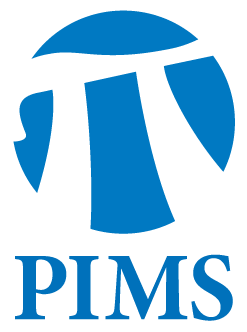## Discrete Math Seminar: Aaron Robertson

• Date: 03/13/2012
• Time: 14:30
Lecturer(s):
Aaron Robertson
Location:

Simon Fraser University

Topic:

Thoughts on the 2-Large Conjecture

Description:

Abstract:

Let $D \\subseteq \\mathbb{Z}^+$. For $r \\in \\mathbb{Z}^+$, we say the $D$ is {\\it $r$-large} if every $r$-coloring of the positive integers admits an arbitrary long monochromatic arithmetic progression with common difference from $D$. Let $\\mathcal{L}_r$ be the class of all $r$-large sets; let $\\mathcal{L} = \\cap_{r=2}^\\infty \\mathcal{L}_r$ and call a set in
$\\mathcal{L}$ {\\it large}. A famous conjecture of Brown, Graham, and Landman states: $\\mathcal{L}_2 \\Longrightarrow \\mathcal{L}$. In this talk, I will survey various approaches to attacking this problem and present some results.

Other Information: